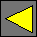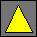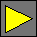April 2002 Draft JavaScript 2.0 Libraries Machine TypesThursday, December 6, 2001

Purpose

Machine types are optional low-level types for use in JavaScript 2.0 programs. Implementations are not required to support these types. These types provide faster, Java-style integer operations that are useful for communicating between JavaScript 2.0 and other programming languages and for performance-critical code. These types are not intended to replace Number and Integer for general-purpose scripting.

Contents

The following types become available:

Type

Unit

Values

sbyte   Integer values between –128 and 127 inclusive, excluding –0.0
byte   Integer values between 0 and 255 inclusive, excluding –0.0
short   Integer values between –32768 and 32767 inclusive, excluding –0.0
ushort    Integer values between 0 and 65535 inclusive, excluding –0.0
int   Integer values between –2147483648 and 2147483647 inclusive, excluding –0.0
uint   Integer values between 0 and 4294967295 inclusive, excluding –0.0
long L Long integer values between –9223372036854775808 and 9223372036854775807 inclusive
ulong UL Long integer values between 0 and 18446744073709551615 inclusive
float F Single-precision IEEE floating-point numbers, including positive and negative zeroes, infinities, and NaN

The above type names are not reserved words. The units are defined using the standard unit facility and may be overridden by the programmer.

8, 16, and 32-bit Integers

The first six types sbyte, byte, short, ushort, int, and uint are all proper subtypes of Integer, which is itself a subtype of Number. A particular number such is a member of multiple types. For example, 3.0 is a member of sbyte, byte, short, ushort, int, uint, Integer, Number, and Object, while –2000.0 is a member of short, int, Integer, Number, and Object. JavaScript does not distinguish between the literals 3 and 3.0 in any way.

All arithmetic operations and comparisons on sbyte, byte, short, ushort, int, and uint values treat them just like they would any other Number values — the operations are performed using full IEEE double-precision arithmetic.

There are no predefined implict or explicit coercions from values of type sbyte, byte, short, ushort, int, or uint other than the coercions predefined on the type Number. The following predefined implicit coercions are applicable when the destination type is sbyte, byte, short, ushort, int, or uint:

• undefined +0.0
• –0.0 +0.0
• long and ulong values within range of the destination type T are converted to equivalent values of type T
• finite integral float values within range of the destination type T are converted to equivalent values of type T
• If the source value x is a finite Integer, finite integral float, long, or ulong not within range of the destination type T and the wrap pragma is in effect, then x is treated modulo |T|, where |int8| = |uint8| = 256, |int16| = |uint16| = 65536, and |int32| = |uint32| = 232.

Note that there are no implicit coercions from NaN or positive or negative infinity to sbyte, byte, short, ushort, int, or uint.

Define explicit coercions — should they truncate (or perhaps round) non-integral values?

64-bit Integers

The types long and ulong represent signed and unsigned 64-bit integers. long and ulong literals are written with the unit L or UL and no exponent or decimal point. Literal values of type long are written as –9223372036854775808L through 9223372036854775807L. Literal values of type ulong are written as 0UL through 18446744073709551615UL.

The types long and ulong are disjoint from Number, so 5L and 5 are different objects, although they compare == to each other. 5L and 5UL are also different objects, although they compare == and === to each other.

Negation, addition, subtraction, and multiplication, and modulo (%) on long and ulong values is exact, and long and ulong values may be mixed in an expression. There are five possible cases depending on the mathematical result x:

• If –9223372036854775808  x  –1, then the result has type long.
• If 0  x  9223372036854775807, then the result has type ulong if at least one operand has type ulong; otherwise, the result has type long.
• If 9223372036854775808  x  18446744073709551615, then the result has type ulong.
• Otherwise, if the wrap pragma is not in effect then an error occurs.
• Otherwise, if at least one operand has type ulong then the result is the ulong value congruent to x modulo 264; otherwise, the result is the long value congruent to x modulo 264.

Division on long and ulong values truncates towards zero to obtain an integer x which is then treated in the above manner.

Division and modulo on long and ulong values can produce the Number values positive infinity or NaN.

The logical operations &, |, and ^ are 64 bits wide if at least one operand is a long or ulong. The result is a ulong if at least one operand is a ulong; otherwise, the result is a long.

The logical shifts <<, >>, and >>> are 64 bits wide if the first operand is a long or ulong. The result is a ulong if the first operand is a ulong; otherwise, the result is a long. >> copies the most significant bit and >>> shifts in zero bits regardless of whether the first operand is a long or ulong.

Arithmetic mixing a long or ulong operand with a Number (or any subtype of Number) or float operand first coerces the Number or float operand to a long value (or ulong if it’s out of range). Comparisons mixing a long or ulong operand with a Number (or any subtype of Number) or float operand compare exact mathematical values without any coercions.

The following predefined implicit coercions are applicable when the destination type is long:

• undefined 0L
• ulong values between 0UL and 9223372036854775807UL are converted to equivalent long values.
• Finite Integer values between –9223372036854775808 and 9223372036854775807 are converted to equivalent long values.
• Finite integral float values between –9223372036854775808F and 9223372036854775807F are converted to equivalent long values.
• If the source value x is a ulong, finite Integer, or finite integral float and the wrap pragma is in effect, then x is treated modulo 264 to produce a long value.

The following predefined implicit coercions are applicable when the destination type is ulong:

• undefined 0L
• long values between 0L and 9223372036854775807L are converted to equivalent ulong values.
• Finite Integer values between –0.0 and 18446744073709551615 are converted to equivalent ulong values.
• Finite integral float values between –0.0F and 18446744073709551615F are converted to equivalent ulong values.
• If the source value x is a long, finite Integer, or finite integral float and the wrap pragma is in effect, then x is treated modulo 264 to produce a ulong value.

Note that there are no implicit coercions from NaN or positive or negative infinity to long or ulong.

A long or ulong value can be implicitly coerced to type Number or Integer. The result is the closest representable Number value using the same rounding as when a string is converted to a number. If the source is 0L or 0UL then the result is +0.0.

Define explicit coercions — should they truncate (or perhaps round) non-integral values?

Single-Precision Floats

The type float represents single-precision IEEE floating-point numbers. float literals are written with the unit F. float infinities and NaN are separate from Number infinities and NaN.

The type float is disjoint from Number, so 5F and 5 are different objects, although they compare == and === to each other.

Arithmetic involving only float values is done using float arithmetic. Arithmetic mixing float and Number (or any subtype of Number) values is done using Number arithmetic. Comparisons mixing a float operand with a Number (or any subtype of Number) operand compare exact mathematical values without any coercions (which in this case is equivalent to first coercing the float to a Number).

The logical operations &, |, ^, <<, >>, and >>> coerce any float operands to type Number before proceeding.

The following predefined implicit coercions are applicable when the destination type is float:

• undefined (NaN)"F"
• Number values (including NaN and the infinities) are converted to the closest representable float values.
• long and ulong values are converted to the closest representable float values.

A float value can be implicitly coerced to type Number. The result is the equivalent Number value.

 Waldemar Horwat Last modified Thursday, December 6, 2001